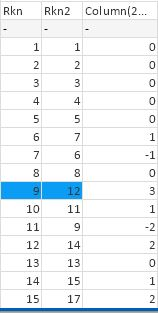# New to QlikView

Discussion board where members can get started with QlikView.

New Contributor III

## Rank for Calculated Expression in Straight Table

Hello all,

I have a project where I have set up a straight table that has two dimensions (newmodeltxt and yeartxt) and numerous calculated expressions.

I want to do several things here:

1. Calculate the Complaint Rate = ((count(DISTINCT(odino))) / (veh_vol))*1000.  This looks good.

2. Calculate the Rank of the Complaint Rate.  I want this calculation to be regardless of any other columns in the table... just a straight sorting of this Complaint Rate column.  In other words, I want to see the highest value of Complaint Rate to be at the top of my table and the lowest value to be at the bottom.  Everything I've tried seems to continue to group by the two dimensions (newmodeltxt and yeartxt)

3. Calculate the change in Rank from the previous month (i.e. using the Month variable to calculate the difference between Rank for the entire time frame, and Rank for the time frame - the most recent month).

Can anyone offer any help with respect to #2 and 3?  Sample file attached:

Thanks!

Noelle

Tags (3)
7 RepliesMVP

## Re: Rank for Calculated Expression in Straight Table

Maybe just use the TOTAL qualifier

=rank(TOTAL (count(DISTINCT(odino)) / (veh_vol))*1000)

New Contributor III

## Re: Rank for Calculated Expression in Straight Table

Stefan,

That worked perfectly - thanks so much for the quick response!

Any ideas about #3?  I need to incorporate the time element (month) to figure how the Rank has changed since the last month.

Something like: =if ( (month) <= (max(month) - 1)), rank(TOTAL (count (DISTINCT (odino) ) / (veh_vol)) * 1,000) - rank(TOTAL (count(DISTINCT(odino)) / (veh_vol))*1000), 0)

Thanks!

NoelleMVP

## Re: Rank for Calculated Expression in Straight Table

Not quite sure I understand. How would your table look like with the given requirement?

New Contributor III

## Re: Rank for Calculated Expression in Straight Table

I'm looking for the left-most column to show the current Rank (your solution above worked for this) and the right-most column to show the change in Rank. Like for sports teams, when you show if they rose or dropped since the last weeks rankings.

I have the Month variable which I hope to use to calculate (Rank during previous month) - (Rank for current month) = change in Rank.

Thanks - Noelle

New Contributor III

## Re: Rank for Calculated Expression in Straight Table

Hi Stefan,

Let me try explaining once more.

In the left-most column, I need the overall Rank calculated based on all of the data, which comes from the formula you provided yesterday - thanks!

In the right-most column, I need the Rank Change.  This is a monthly report, and each row of data has an associated date.  I need to calculate the rank based on all the data excluding the most recent month's data; i.e. where Month = max(Month).  In other words, the overall Rank from the previous monthly report.  I could then subtract The Overall Ranking from the Previous Month Ranking to find the change in rank from the previous month to this month.

Any ideas would be greatly appreciate.

Thanks!  Noelle

New Contributor III

## Re: Rank for Calculated Expression in Straight Table

Hi QlikView community -

Circling back to this thread to see if anyone else has any ideas about calculating the Rank for a subset of the data (ignoring the most recent month).

Thanks!

Noelle

New Contributor II

## Re: Rank for Calculated Expression in Straight Table

Hi Noelle,Like this?I use this expression

Rkn:

Rank(if(Count(distinct New_Corp)=1,\$(MAT_ACT)))

Rkn2:

Rank(if(Count(distinct New_Corp)=1,\$(MAT_PY)))

and the 3rd column is the difference:

Rank(if(Count(distinct New_Corp)=1,\$(MAT_PY)))-Rank(if(Count(distinct New_Corp)=1,\$(MAT_ACT)))

Where MAT_ACT is my variable for the current period

Where MAT_PY is my variable for the current period

and New_Corp the dimension

Kind Regards